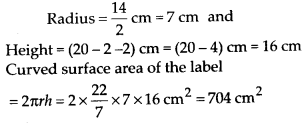## MP Board Class 8th Maths Solutions Chapter 11 Mensuration Ex 11.3

Question 1.
There are two cuboidal boxes as shown in the adjoining figure. Which box requires the lesser amount of material to make?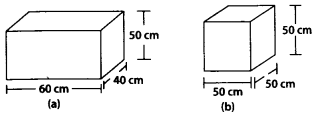Solution:
Surface area of box (a) = 2(lb + bh + hl)
= 2(60 × 40 + 40 × 50 + 50 × 60)
= 2(2400 + 2000 + 3000)
= 2 × 7400 = 14800 cm2
Surface area of box (b) = 6 × (side)2 = 6 × 502 = 15000 cm2
Hence, box (a) required less amount of material than box (b) to make.Question 2.
A suitcase with measures 80 cm × 48 cm × 24 cm is to be covered with a trapaulin cloth. How many metres of trapaulin of width 96 cm is required to cover 100 such suitcases?
Solution:
Let l, b and h be the length and breadth and height of the suitcase respectively.
⇒ l = 80 cm, b = 48 cm, h = 24 cm
Total surface area of suitcase = 2(lb + bh + hl)
= 2(80 × 48 + 48 × 24 + 24 × 80)
= 2(3840 + 1152 + 1920) cm2 = 2(6912) cm2 = 13824 cm2
Area of cloth required for 1 suitcase = Area of 1 suitcase
⇒ l × 96 = 13824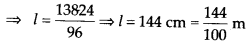Length required for 100 suitcases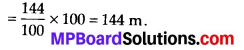Question 3.
Find the side of a cube whose surface area is 600 cm2.
Solution:
Surface area of cube = 6 × (side)2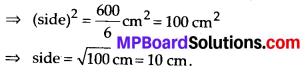Question 4.
Rukhsar painted the outside of the cabinet of measure 1 m × 2 m × 1.5 m. How much surface area did she cover if she painted all except the bottom of the cabinet.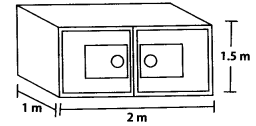Solution:
Rukhsar painted all the cabinet except the bottom means she painted 4 walls and 1 top. Let l = 1 m, b = 2 m, h = 1.5 m be the length, breadth and height of cabinet respectively.
Area of painted cabinet
= (lb + bh + bh + lh + lh)
= [l × 2 + 2 × 1.5 + 2 × 1.5 + l × 1.5 + l × 1.5]m2
= [2 + 3.0 + 3.0 +1.5 +1.5] m2 = 11 m2.Question 5.
Daniel is painting the walls and ceiling of a cuboidal hall with length, breadth and height of 15 m, 10 m and 7 m respectively. From each can of paint 100 m2 of area is painted. How many cans of paint will she need to paint the room?
SOlution:
Daniel is painting 4 walls and 1 ceiling.
∴ Total painted area = Area of 4 walls + Area of ceiling
Let l = 15, b = 10 and h = 7 be the length, breadth and height of the hall respectively. = 2 × h(l + b) + lb = {2 × 7 (15 +10) + (15 × 10)} m2
= {14 (25) +150} m2 = {350 +150} m2 = 500 m2
If 100 m2 of area is painted with one can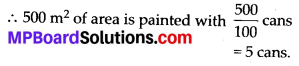Question 6.
Describe how the two figures given below are alike and how they are different. Which box has larger lateral surface area?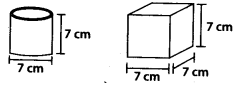Solution:
Alike: They both have same height. Different: Their shapes are different one is cylinderical and other is cubical box.
Let r and h be the radius and height of the cylinder.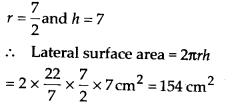Let the side of cube be a = 7 cm
⇒ Lateral surface area = 4(a)2 = 4(7)2 cm2 = 196 cm2
∴ Cubical box has larger lateral surface area.

Question 7.
A closed cylindrical tank of radius 7 m and height 3 m is made from a sheet of metal. How much sheet of metal is required ?
Solution:
Let r and h be the radius and height of the closed cylinder.
Total surface area = 2πr(r + h)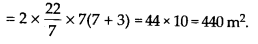Question 8.
The lateral surface area of a hollow cylinder is 4224 cm2. It is cut along its height and formed a rectangular sheet of width 33 cm. Find the perimeter of rectangular sheet ?
Solution:
Let r and h be the radius and height of the hollow cylinder and l be its lateral surface area.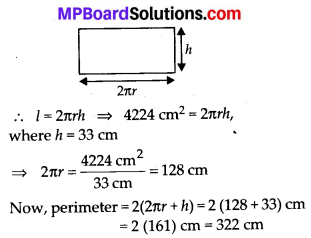Question 9.
A road roller takes 750 complete revolutions to move once over to level a road. Find the area of the road if the diameter of a road roller is 84 cm and length is 1 m.
Solution:
Area covered in 1 revolution = curved surface area of cylinder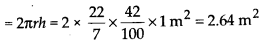∴ Area covered in 750 revolutions = 2.64 × 750 m2 = 1980 m2Question 10.
A company packages its milk powder in cylindrical container whose base has a diameter of 14 cm and height 20 cm. Company places a label around the surface of the container (as shown in figure). If the label is placed 2 cm from top and bottom, what is the area of the label.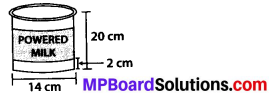Solution: